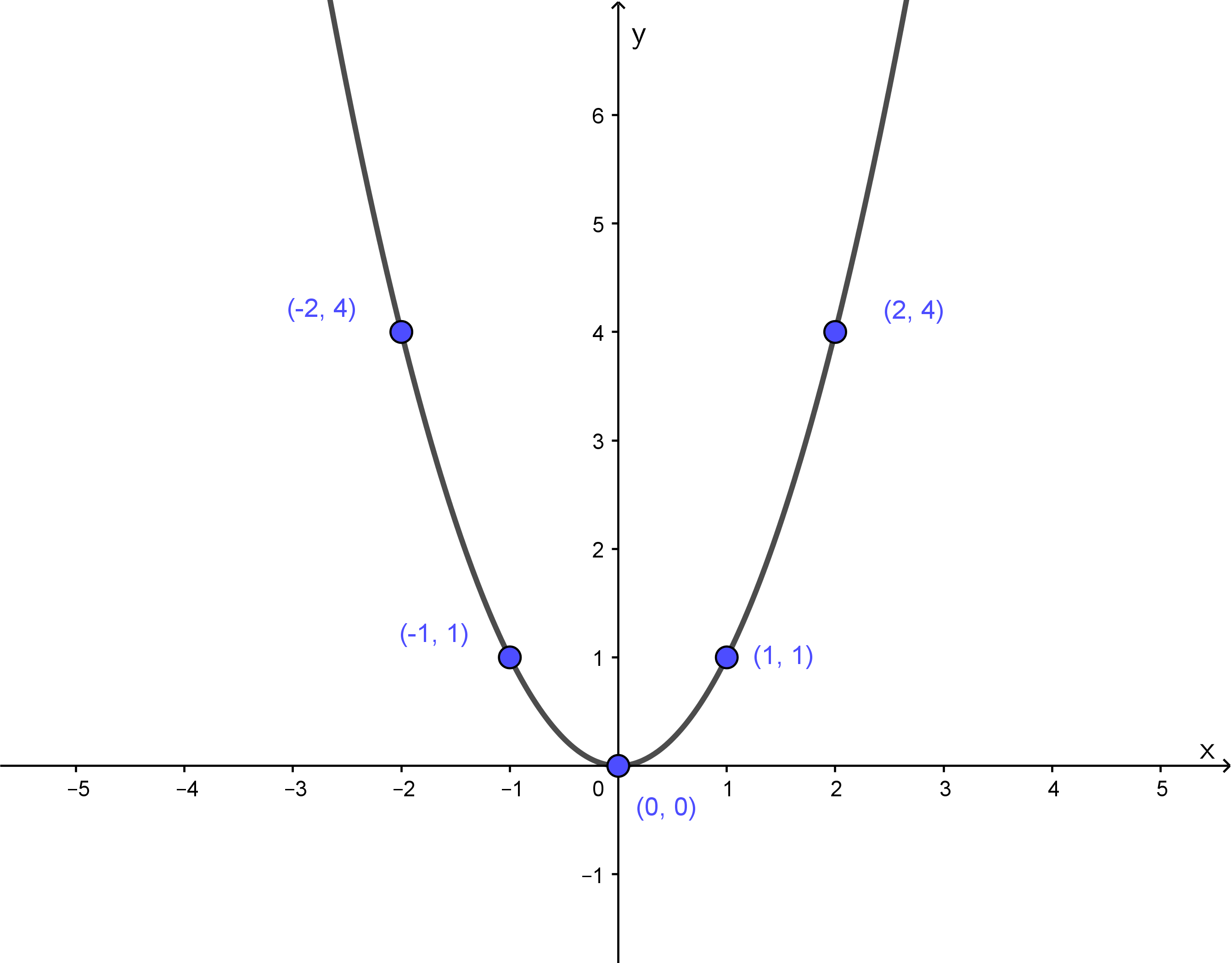# Sections 2.6 - Graphing Special Parabolas

Section Objectives

1. Graph parabolas whose equations have the form $y=ax^2$.
2. Graph parabolas whose equations have the form $y=ax^2+c$.

### Parabolas

The graph of a quadratic equation of the form $y=ax^2+bx+c$ is called a parabola. Parabolas are examples of more general conic sections. A parabola is a smooth U-shaped curve. The turning point of the U is called the vertex, and parabolas are symmetric about the line through the vertex.

The parabola described by $y=x^2$ is a graph you should become familiar with. The graph is a smooth U shape, opening upward, with its vertex at $(0,0)$.The standard parabola can be transformed in a number of ways:

• The graph of $y=-x^2$ is flipped about the $x$-axis.
• The graph of $y=ax^2$ with $a>1$ is vertically stretched.
• The graph of $y=ax^2$ with $0 is vertically compressed.
• The graph of $y=x^2+c$ is shifted up or down depending on the sign of $c$. The vertex is at $(0,c)$.

By thinking about these transformations and plotting a few points, it is easy to graph basic parabolas.

#### Examples

• Consider the parabola described by $y=2x^2$. Make a table of points satisfying the equation and sketch the graph.

• Consider the parabola described by $y=-3x^2$. Make a table of points satisfying the equation and sketch the graph.

• Consider the parabola described by $y=2x^2-1$. Make a table of points satisfying the equation and sketch the graph.